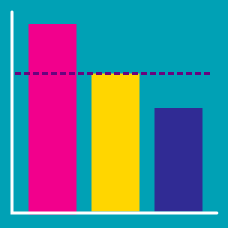Discrete Mathematics

# Linearity of Expectation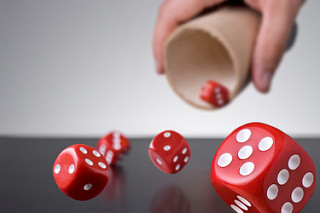If you roll a six-sided die, the expected value for the number rolled is $$3.5.$$ If you roll 5 six-sided dice, what is the expected value for the sum of the numbers rolled?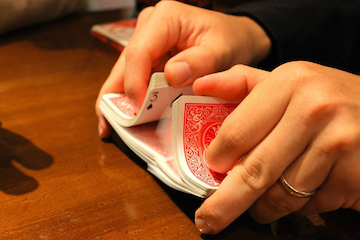A standard deck of 52 cards is shuffled randomly, and then the cards are flipped over one-by-one. If the ace of spades is card number $$S$$ and the ace of hearts is card number $$H$$, what is the expected value of $$S+H?$$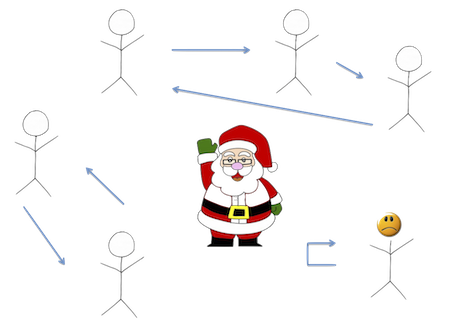Six friends are doing a "secret santa gift exchange". Their names are placed into a hat, and each person chooses a name randomly (without replacement) from the hat.

However, this leads to the possibility that someone chooses their own name and doesn’t get a gift! What is the expected value for the number of people who will choose their own name?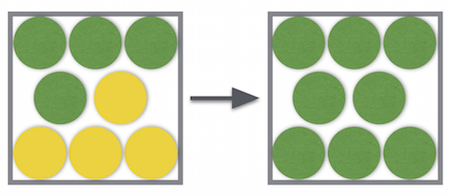Patrick has a box with 4 yellow balls and 4 green balls. He randomly pulls out a ball, and if it is yellow, he paints it green. He then puts it back in the box and continues this process until all of the balls are green. Find the expected value for the number of times Patrick will pull out a ball.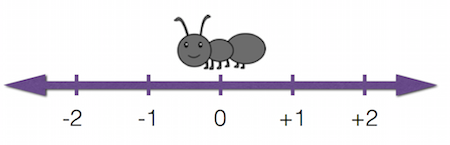Andy the Ant is on a number line at the location 0. Each minute, he travels 1 unit to the right with probability $$\frac{3}{4}$$ and 1 unit to the left with probability $$\frac{1}{4}$$. What is the expected value for Andy’s location after one hour?

×

Problem Loading...

Note Loading...

Set Loading...